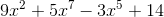# TExES Mathematics 7-12 (235): Practice & Study Guide Final Exam

Free Practice Test Instructions:

Choose your answer to the question and click 'Continue' to see how you did. Then click 'Next Question' to answer the next question. When you have completed the free practice test, click 'View Results' to see your results. Good luck!

#### Question 2 2. Describe the behavior of this function: as x increases, what happens to y?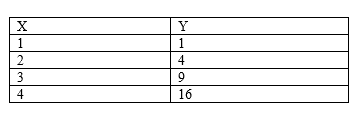#### Question 3 3. Use the given graph and formula to solve for z.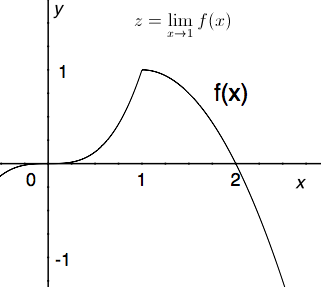#### Question 5 5. If the following graph is f(x), then which graph below could be f'(x)?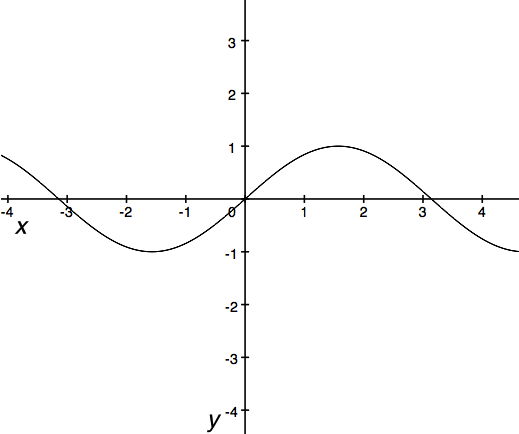#### Question 7 7. For the following function, what is the amplitude?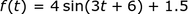#### Question 13 13. What is the average rate of change for the interval with endpoints A and B?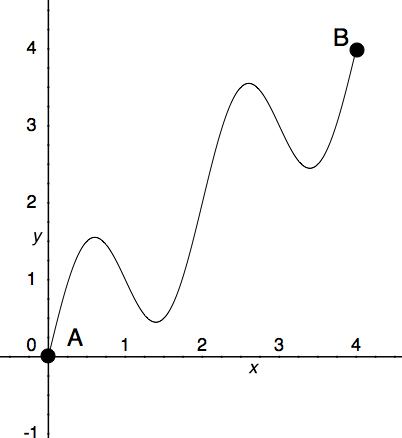#### Question 14 14. Which of the following integrals is equal to the following summation over the continuous region x=a to x=b?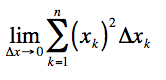#### Question 15 15. What is the degree of the following polynomial?